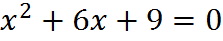# Equations

## Performing Operations on Equations

An equation is like a balanced scale. If we remove or add weight to one side alone, it’s no longer balanced, and hence no longer an equation. We can freely alter the weight on one side if we make the same adjustment on the other, keeping the balance.

What is y in this equation?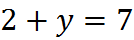We want to know what y is, so we want to “isolate” y (get it by itself) on one side of the equal sign.

First subtract 2 from both sides.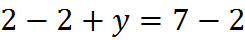After we subtracted, y is by itself and we can see the value of y: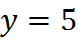Let’s try another example, solving for x: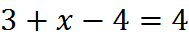Add 4 to each side to get rid of the -4.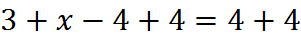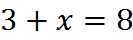Subtract 3 from each side of the equation.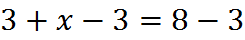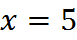## Types of Equations

The GMAT will not explicitly require you to distinguish different types of equations, but, in practice, you will have to be able to do so in order to solve them all.

A linear equation has variables that are not multiplied by each other or taken to any exponent. For example,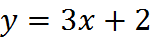is a linear equation, because the variables x and y are not multiplied by each other or taken to an exponent. And y = 3x + 2 is the equation of a line in the xy-plane (in the common form y = mx + b), so “linear” is not a bad name for this type of equation.

Linear equations often appear on the GMAT in groups, in which case they are “systems” of linear equations. It’s a “system” just because it has more than one equation. On many GMAT word problems, we can solve by translating the descriptions given into a system of equations and then solving the system of equations.

A quadratic equation has a squared variable. For example,